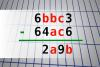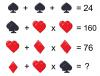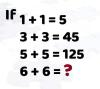BRAIN TEASERSBrain Teasers User Profile

# Hamey Idris

rank
520
points
3
See full ranking list
short ranking list
 518 ASHANAND BAIRWA 3 519 Faisal Nadeem 3 520 Hamey Idris 3 521 Ramon Vidals 3 522 mark cevaal 3
 Find number abc If 6bbc3 - 64ac6 = 2a9b find number abc. Multiple solutions may exist.Can you replace the question mark with a number? MATH PUZZLE: Can you replace the question mark with a number?Mathematical Puzzle: IF 1+1=... Mathematical Puzzle: IF 1+1=5, 3+3=45 and 5+5=125 THEN 6+6=?